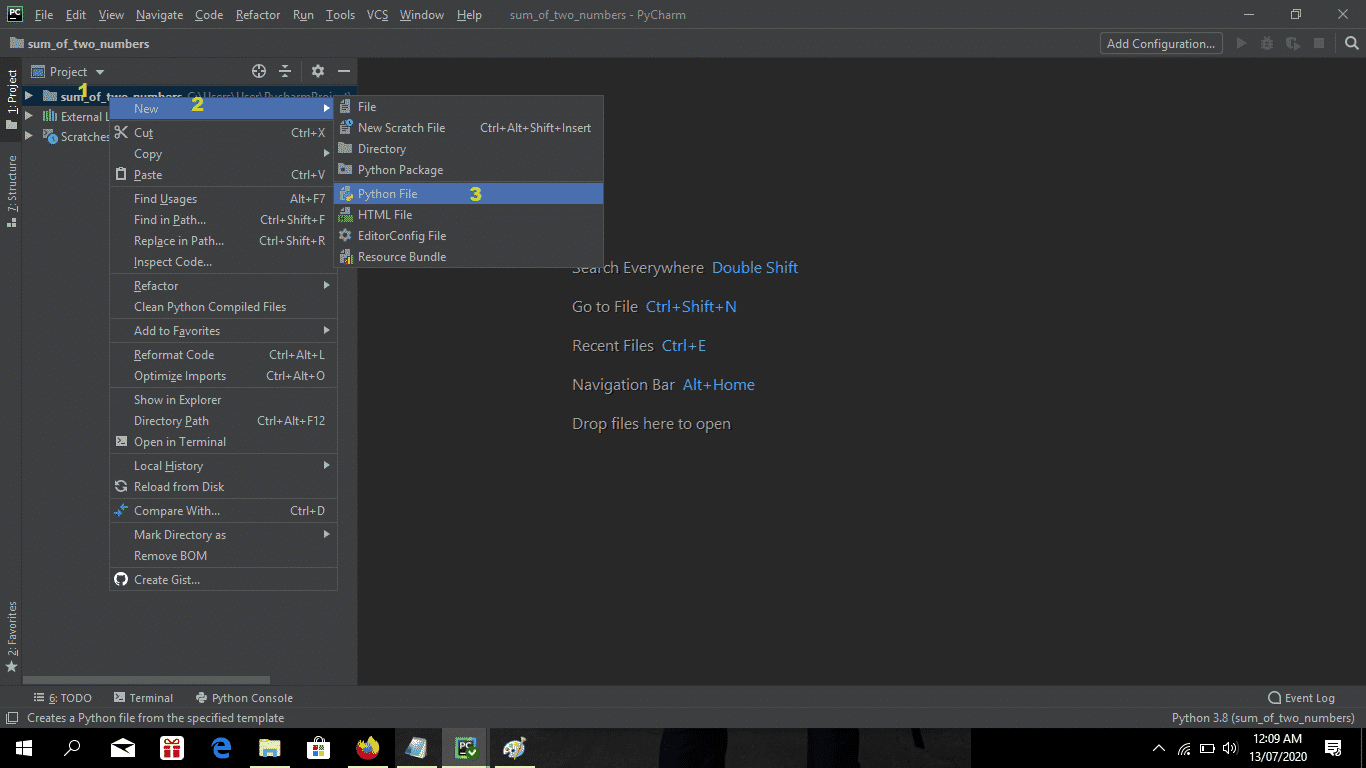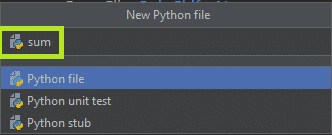# Sum of Two Numbers In Python With Example

This article is an easy way on how to Print Sum of Two Numbers In Python. This code will dynamically sum the 2 given numbers when user click on the calculate button.

## How To Print Sum Of Two Numbers using Python : Project Information

The code use tkinter module to create a layout and widgets that may call a specific python functions.

We will be the usage of Python programming language because it’s far widely used as an advance-degree programming language for a general approach to the developer. This tutorial also includes the downloadable Sum of 2 Numbers In Python source code.

To start creating on How To Print Sum Of Two Numbers, make sure that you have Pycharm IDE installed in your computer.

By the way if you are new to python programming and you don’t know what would be the the Python IDE to use, I have here a list of Best Python IDE for Windows, Linux, Mac OS that will suit for you. I also have here How to Download and Install Latest Version of Python on Windows.

## Steps on How To Print Sum Of Two Numbers In PythonWith Source Code

How To Print Sum Of Two Numbers With Source Code

• Step 1: Create a project name.

First step open Pycharm IDE and click “file” after that create a project name and then click “create” button.• Step 2: Create python file name.

Second step “right click” your project name folder and choose new and then click “python file“.• Step 3: Name your python file.

Third step name your python file and click “enter” to start creating on How To Print Sum Of Two Numbers• Step 4: The actual code.

Fourth step is the actual coding on How To Print Sum Of Two Numbers.

## Python Program To Add Two Numbers Code Explanations

### 1. Import Tkinter

The code given below is for importing tkinter modules.

``````from tkinter import *
from tkinter import messagebox``````

Importing the tkinter modules.

### 2. GUI Design

The code given below is for the GUI design.

``````        def check():

self.label1 = Label(self, text="Enter Number 1" ,  fg = 'black', font=("courier-new", 18, 'bold'))
self.label1.place(x=280, y=180)
self.num1 = Entry(self, textvariable=self.a,bg='light blue', width=45)
self.num1.place(x=480, y=190)
self.label2 = Label(self, text="Enter Number 2" , fg = 'black', font=("courier-new", 18, 'bold'))
self.label2.place(x=280, y=250)
self.num2 = Entry(self, textvariable=self.b,bg='light blue', width=45)
self.num2.place(x=480, y=255)
self.butt = Button(self, text="Calculate",bg ='green', font=10, width=8, command=chex).place(x=580, y=300)
self.label3 = Label(self, text="Sum of Two Numbers", fg='black', font=("courier-new", 24, 'bold'))
self.label3.place(x=380, y=30)``````

This module which is the design of this project using Graphical User Interface (GUI).

### 3. Calculate Two Numbers

The code given below is for calculating the two numbers.

``````        def chex():
if not self.num1.get() and not self.num2.get():
else:
res = int(self.num1.get()) + int(self.num2.get())
messagebox.showinfo('Sum of Two Numbers', "The answer is: %d" % (res))
self.num1.set = ""
self.num2.set = ""``````

This module which calculate the two input number in addition.

### Complete Source Code

``````from tkinter import *
from tkinter import messagebox

#creating window
class Lib(Tk):
def __init__(self):
super().__init__()
self.a = StringVar()
self.b = StringVar()
self.maxsize(1200, 700)
self.minsize(1200, 700)
self.configure(bg="white")
self.title("Sum of Two Numbers by: IT SOURCECODE")

#verifying input
def chex():
if not self.num1.get() and not self.num2.get():
else:
res = int(self.num1.get()) + int(self.num2.get())
messagebox.showinfo('Sum of Two Numbers', "The answer is: %d" % (res))
self.num1.set = ""
self.num2.set = ""

def check():

self.label1 = Label(self, text="Enter Number 1" ,  fg = 'black', font=("courier-new", 18, 'bold'))
self.label1.place(x=280, y=180)
self.num1 = Entry(self, textvariable=self.a,bg='light blue', width=45)
self.num1.place(x=480, y=190)
self.label2 = Label(self, text="Enter Number 2" , fg = 'black', font=("courier-new", 18, 'bold'))
self.label2.place(x=280, y=250)
self.num2 = Entry(self, textvariable=self.b,bg='light blue', width=45)
self.num2.place(x=480, y=255)
self.butt = Button(self, text="Calculate",bg ='green', font=10, width=8, command=chex).place(x=580, y=300)
self.label3 = Label(self, text="Sum of Two Numbers", fg='black', font=("courier-new", 24, 'bold'))
self.label3.place(x=380, y=30)

check()

Lib().mainloop()``````